How Cheenta works to ensure student success?
Explore the Back-Story

# ISI Entrance 2006 - B.Math Subjective Paper| Problems & SolutionsHere, you will find all the questions of ISI Entrance Paper 2006 from Indian Statistical Institute's B. Math Entrance. You will also get the solutions soon of all the previous year problems.

Problem 1:

Bishops on a chessboard move along the diagonals ( that is, on lines parallel to the two main diagonals). Prove that the maximum number of non-attacking bishops on an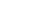chessboard is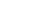. (Two bishops are said to be attacking if they are on a common diagonal).

Problem 2:

Prove that there is no non-constant polynomial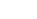with integer coefficients such that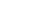is a prime number for all positive integers n.

Problem 3:

Find all roots of the equation :-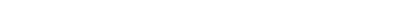Problem 4:

Let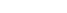be a function that is a function that is differentiable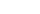times for some positive integer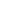. The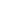derivative of f is denoted by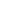. Suppose-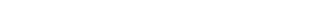Prove that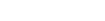for some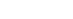Problem 5:

A domino is a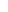byrectangle. For what integers m and n can we cover an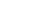rectangle with non-overlapping dominoes?

Problem 6:

You are standing at the edge of a river which is 1 km wide. You have to go to your camp on the opposite bank. The distance to the camp from the point on the opposite bank directly across you is 1 km. You can swim at 2 km/hr and walk at 3 km-hr. What is the shortest time you will take to reach your camp? (Ignore the speed of the river and assume that the river banks are straight and parallel).

Problem 7:

In a triangle ABC , D is a point on BC such that AD is the internal bisector of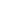. Now Suppose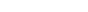and CD=AB . Prove that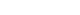Problem 8:

Let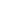be the set of all integers k,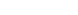, such that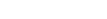. What is the arithmetic mean of the integers in?

Here, you will find all the questions of ISI Entrance Paper 2006 from Indian Statistical Institute's B. Math Entrance. You will also get the solutions soon of all the previous year problems.

Problem 1:

Bishops on a chessboard move along the diagonals ( that is, on lines parallel to the two main diagonals). Prove that the maximum number of non-attacking bishops on anchessboard is. (Two bishops are said to be attacking if they are on a common diagonal).

Problem 2:

Prove that there is no non-constant polynomialwith integer coefficients such thatis a prime number for all positive integers n.

Problem 3:

Find all roots of the equation :-Problem 4:

Letbe a function that is a function that is differentiabletimes for some positive integer. Thederivative of f is denoted by. Suppose-Prove thatfor someProblem 5:

A domino is abyrectangle. For what integers m and n can we cover anrectangle with non-overlapping dominoes?

Problem 6:

You are standing at the edge of a river which is 1 km wide. You have to go to your camp on the opposite bank. The distance to the camp from the point on the opposite bank directly across you is 1 km. You can swim at 2 km/hr and walk at 3 km-hr. What is the shortest time you will take to reach your camp? (Ignore the speed of the river and assume that the river banks are straight and parallel).

Problem 7:

In a triangle ABC , D is a point on BC such that AD is the internal bisector of. Now Supposeand CD=AB . Prove thatProblem 8:

Letbe the set of all integers k,, such that. What is the arithmetic mean of the integers in?

This site uses Akismet to reduce spam. Learn how your comment data is processed.

### One comment on “ISI Entrance 2006 - B.Math Subjective Paper| Problems & Solutions”

1.Spandan says:

Problems 1 and 3 requested

### Knowledge Partner